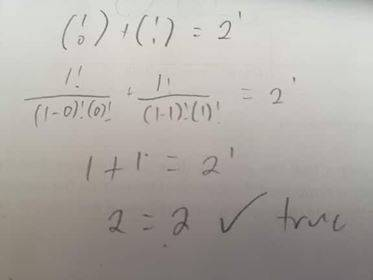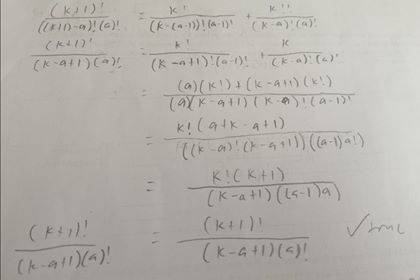# Proof by mathematical induction

• Rainbow Cupcake
In summary, the conversation is discussing how to prove the mathematical statement (n 0) + (n 1) + (n 2) + ... + (n n) = 2^n using mathematical induction. The falling factorial notation (n n) is mentioned but it is clarified that the problem is likely referring to binomial coefficients instead. The conversation also mentions a formula for binomial coefficients and how it can be used to help prove the statement. However, it is pointed out that the statement is not true for falling factorials.f

#### Rainbow Cupcake

Summary:: prove that (n 0) + (n 1) + (n 2) + ... + (n n) = 2^n is true using mathematical induction.
note that (n n) is a falling factorial

Hello! I have trouble dealing with this problem:

Mod note: Thread moved from math technical section, so is missing the homework template.
Prove that (n 0) + (n 1) + (n 2) + ... + (n n) = 2^n is true using mathematical induction.
note that (n n) is a falling factorial. I have made some progress with this problem but I got eventually got stuck.

progress:

let n=1 (please see attached photo)Therefore it is true for n=1

Then we assume that (k 0) + (k 1) + ... + (k k) = 2^k is true. This could also be written as
(k 0) + (k 1) + ... + (k k-1) + (k k) = 2^k.

Prove that (k+1 a) = (k a-1) + (k a) (pls see attached photo for progress)After this step, i got stuck.

Thank you!

Last edited by a moderator:
•Delta2
If you are stuck trying to show that:
$$\binom{k+1}{a} = \binom{k}{a} + \binom{k}{a-1}$$
Try adding the two terms on the right-hand side and see if you can get the term on the left-hand side.

•Infrared and Delta2
Remember the binomial formula? It says $(a+b)^{n}=a^{n}+\begin{pmatrix} n \\ 1\\ \end{pmatrix}a^{n-1}b+\begin{pmatrix} n \\ 2\\ \end{pmatrix}a^{n-2}b^{2}+...+b^{n}$
Now put a=1 and b=1 into that formula and see what happens.

•WWGD
@Svein here ##\binom{n}{k}:=n(n-1)\cdots (n-(k-1))=\tfrac{n!}{(n-k)!}## is the falling factorial, the binomial formula would read (in terms of the falling factorial) ##(a+b)^n=\sum_{k=0}^n \tfrac{1}{k!}\binom{n}{k}a^{n-k}b^{k}## but this doesn't help here.

Here is a problem: @Rainbow Cupcake is using the definition of binomial coefficients ##\binom{n}{k}=\tfrac{n!}{(n-k)!k!}## in their work when it was clearly stated that ##\binom{n}{k}=\tfrac{n!}{(n-k)!}## is the falling factorial. OP clear this up: which is it ##\binom{n}{k}##, failing factorial or binomial coefficients? Then we have hope.

I did check and the thing you wanted to prove ##\binom{k+1}{a} =\binom{k}{a-1} + \binom{k}{a}## as it turns out, isn't true for falling factorials if that helps...

@Svein here ##\binom{n}{k}:=n(n-1)\cdots (n-(k-1))=\tfrac{n!}{(n-k)!}## is the falling factorial, the binomial formula would read (in terms of the falling factorial) ##(a+b)^n=\sum_{k=0}^n \tfrac{1}{k!}\binom{n}{k}a^{n-k}b^{k}## but this doesn't help here.

Here is a problem: @Rainbow Cupcake is using the definition of binomial coefficients ##\binom{n}{k}=\tfrac{n!}{(n-k)!k!}## in their work when it was clearly stated that ##\binom{n}{k}=\tfrac{n!}{(n-k)!}## is the falling factorial. OP clear this up: which is it ##\binom{n}{k}##, failing factorial or binomial coefficients? Then we have hope.

I did check and the thing you wanted to prove ##\binom{k+1}{a} =\binom{k}{a-1} + \binom{k}{a}## as it turns out, isn't true for falling factorials if that helps...
The context shown in this problem and the notation used suggest to me that this isn't about the falling factorial, but rather, binomial coefficients.

This article on the Wolfram site - https://mathworld.wolfram.com/FallingFactorial.html - show this notation for the falling factorial: ##(x)_n##, defined as ##x(x - 1)(x - 2)\dots(x - (n - 1))##.

This will be of no help in proving that ##\binom n 0 + \binom n 1 + \binom n 2 + \dots + \binom n n = 2^n##

•berkeman and PeroK